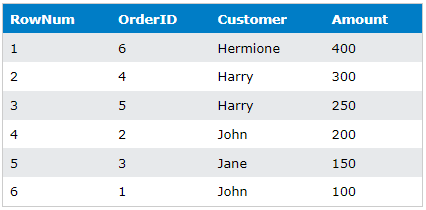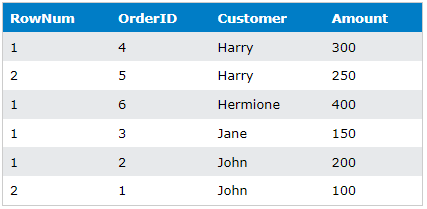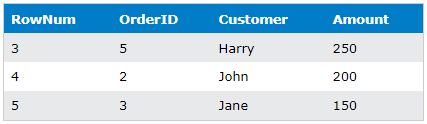﻿ SQL ROW_NUMBER

# SQL ROW_NUMBER

## Understanding how to handle rows and their ordering in SQL is vital for any data analyst or data scientist. The SQL ROW_NUMBER is one of the most useful functions when dealing with such tasks.

You can start practicing the SQL SERVER ROW_NUMBER function for free by creating a free account on FreeASPHosting.net and creating a free SQL Server Database.

### KEY TAKEAWAYS:

1. The SQL ROW_NUMBER function provides a unique row number for each row based on the ordering.
2. ROW_NUMBER() is used with the OVER keyword and can be combined with PARTITION BY to divide the result set into partitions.
3. SQL ROW_NUMBER is useful for SQL pagination, allowing you to fetch a specific range of records from a large result set.
4. ROW_NUMBER OVER PARTITION BY is also helpful when you want to find the highest or lowest value within each group of rows.

## SQL ROW_NUMBER

The SQL ROW_NUMBER function is a built-in window function of SQL Server that provides a unique row number for each row, based on the order that the rows are sorted in the SELECT statement. The numbering starts at 1 and increments by 1 for each row. This can be very useful when dealing with large datasets where a unique identifier isn't present or needs to be generated.

### SQL ROW_NUMBER Syntax

The syntax for the SQL ROW_NUMBER function is straightforward. It is used with the OVER keyword, and the complete syntax looks like this:

``````ROW_NUMBER() OVER (
[PARTITION BY column_1, column_2, ..., column_n]
ORDER BY column_1 [ASC|DESC], column_2 [ASC|DESC], ..., column_n [ASC|DESC]
)

``````
1. ROW_NUMBER() is the function name.
2. “SQL OVER clause” is used to define the window or set of rows over which the ROW_NUMBER function operates.
3. “PARTITION BY” clause is optional and is used to divide the result set into partitions (or groups) that the ROW_NUMBER() function is applied to. If not specified, the function treats the whole result set as a single partition.
4. “ORDER BY” is an optional clause and specifies the order in which the rows have to be numbered or processed within each partition. In other words it tells SQL Server to sort the rows in each partition before assigning row numbers.

## ROW_NUMBER OVER in SQL

To better understand the function of ROW_NUMBER OVER in SQL, let's consider an example. Suppose we have a table named 'Orders' with the following data:If we want to assign a unique row number to each row regardless of any criteria, we use the SQL ROW_NUMBER() function as follows:

``````SELECT
ROW_NUMBER() OVER (ORDER BY Amount DESC) AS RowNum,
OrderID,
Customer,
Amount
FROM Orders;

``````

The resulting table of ROW_NUMBER OVER SQL will look like this:It's noteworthy that the SQL ROW_NUMBER function numbers the rows according to the 'Amount' column in descending order, which we specified in the ORDER BY clause.

## ROW_NUMBER OVER PARTITION BY

However, if we want to reset the row number for each different `Customer` by dividing the result set into partitions, we use the ROW_NUMBER OVER PARTITION BY clause. When you add the PARTITION BY clause to the window function, it will create a 'partition' of rows with the same values, and the row_number function will restart the count for each partition.

``````SELECT
ROW_NUMBER() OVER (PARTITION BY Customer ORDER BY Amount DESC) AS RowNum,
OrderID,
Customer,
Amount
FROM Orders;

``````

In this case, the SQL ROW_NUMBER result set using PARTITION BY would look like this:Here, the ROW_NUMBER OVER PARTITION BY function first divides the rows based on the 'Customer' and then orders them by 'Amount' within each Customer.

## SQL Pagination

SQL ROW_NUMBER is particularly useful when dealing with SQL pagination. Pagination is a mechanism to handle the large result set in manageable chunks or sets. ROW_NUMBER SQL can be used to fetch a range of records, which is perfect for paging purposes.

Let's assume we want to select records from 3rd to 5th. We can use the SQL ROW_NUMBER function to accomplish this:

``````SELECT * FROM (
SELECT
ROW_NUMBER() OVER (ORDER BY Amount DESC) AS RowNum,
OrderID,
Customer,
Amount
FROM
Orders
) AS MyOrders
WHERE RowNum BETWEEN 3 AND 5;

``````

This SQL Pagination query using ROW_NUMBER will return the following records:## Find the Highest Value of the Group Using SQL ROW_NUMBER

There are scenarios where you might want to group by some column and select the row with the highest (or lowest) value from each group. This is where ROW_NUMBER OVER PARTITION BY comes in handy.

Assuming we want to select the order with the highest Amount for each customer, we can use:

``````SELECT OrderID,
Customer,
Amount
FROM   (
SELECT OrderID,
Customer,
Amount,
ROW_NUMBER()
OVER (
partition BY Customer
ORDER BY Amount DESC)
AS RowNum
FROM
Orders
) AS subquery
WHERE  RowNum = 1;

``````

Our output for the above SQL Server ROW_NUMBER query would be:## CTE with SQL ROW_NUMBER

A common table expression (CTE) can be thought of as a temporary result. TSQL ROW_NUMBER is often used in conjunction with CTE to solve complex problems.

Let's say we want to achieve the same above results with CTE for finding the highest value from each group. We can use a CTE along with ROW_NUMBER in SQL to accomplish this:

``````WITH orderCTE
AS (
SELECT
OrderID,
Customer,
Amount,
ROW_NUMBER()
OVER (
partition BY Customer
ORDER BY Amount DESC)
AS RowNum
FROM
Orders
)
SELECT OrderID,
Customer,
Amount
FROM   ordercte
WHERE  RowNum = 1;

``````

### Final words for SQL ROW_NUMBER

The SQL ROW_NUMBER function is a highly useful function in SQL Server for tasks that require dealing with row numbers or pagination. Whether it's simply adding a sequence number to rows, dividing data into partitions, dealing with large datasets that require pagination, or tackling complex tasks involving CTEs, the SQL ROW_NUMBER function should be one of your go-to tools.